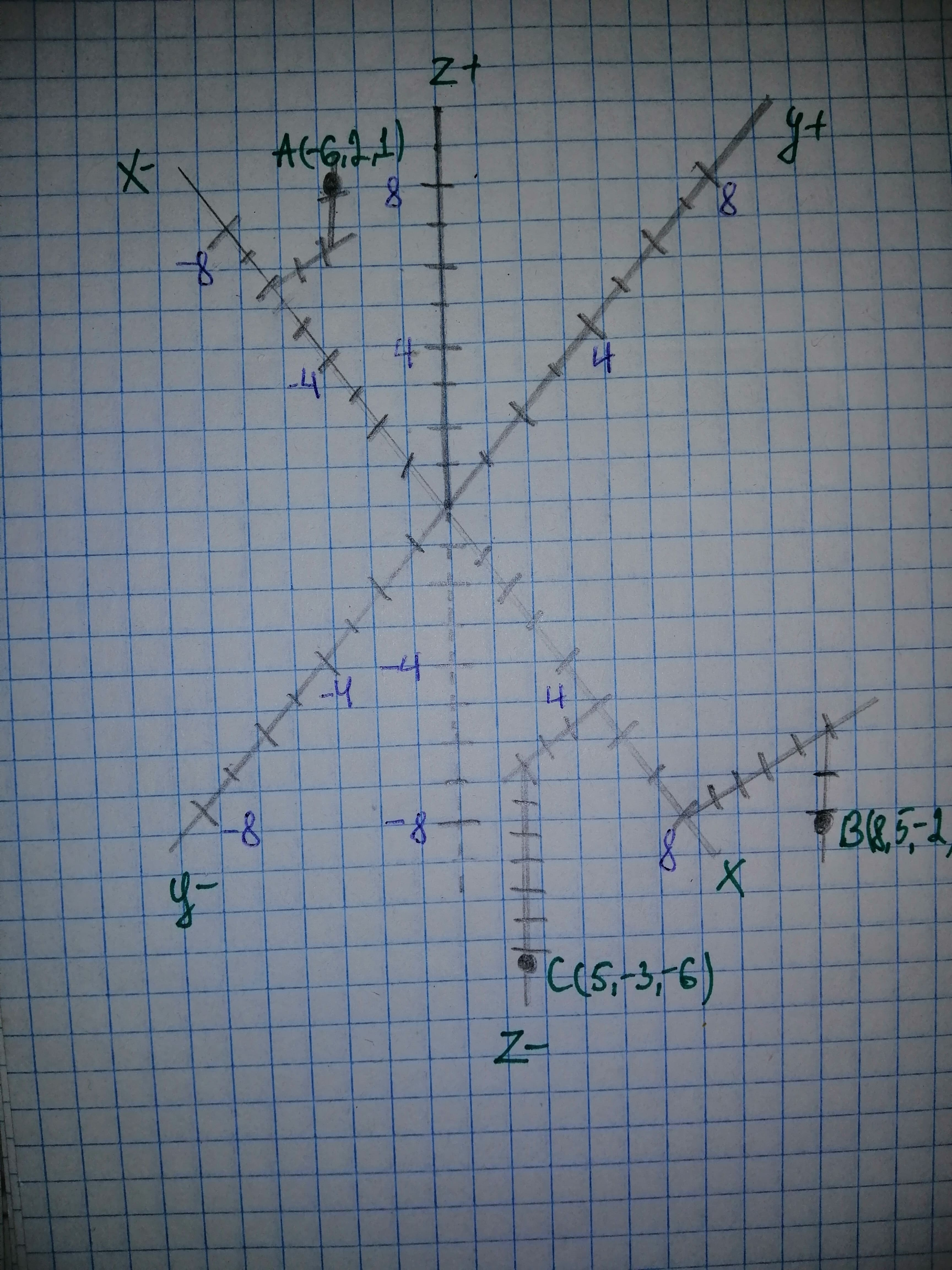# To Sketch: The points on three - axis Cartesian coordinate system. Point A: $\left(-6,\ 2,\ 1\right)$ Point B: $\left(-6,\ 2,\ 1\right)$ Point C: $\left(5,\ -3,\ -6\right)$Wotzdorfg 2021-01-02 Answered
To Sketch: The points on three - axis Cartesian coordinate system.
Point A:
Point B:
Point C:
You can still ask an expert for help

• Questions are typically answered in as fast as 30 minutes

Solve your problem for the price of one coffee

• Math expert for every subject
• Pay only if we can solve itSchepperJ

The three axes Cartesian coordinate system point is to be considering in space. A point is lacated from origin by three-dimension x, y and z.
The dimensions x, y and z are perpendicular to eqch other. The value of x given first, then value of y and then value of z.